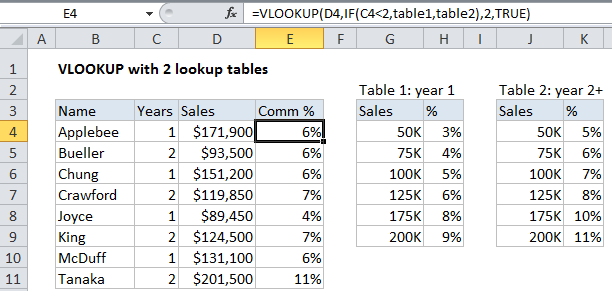## Excel Office

Excel How Tos, Tutorials, Tips & Tricks, Shortcuts

# VLOOKUP with 2 lookup tables in Excel

This tutorial shows how to calculate VLOOKUP with 2 lookup tables in Excel using the example below;

## Formula

`=VLOOKUP(value,IF(test,table1,table2),col,match)`## Explanation

To use VLOOKUP with a variable table array, you can use the IF function inside VLOOKUP to control which table is used.

In the example shown the formula in cell E4 is:

`=VLOOKUP(D5,IF(C4<2,table1,table2),2,TRUE)`

This formula uses the number of years a salesperson has been with a company to determine which commission rate table to use.

Worked Example:   Calculate grades with VLOOKUP in Excel

### How this formula works

Working from the inside out, the IF function in this formula, which is entered as the “table_array” argument in VLOOKUP, runs a logical test on the value in column C “Years”, which represents the number of years a salesperson has been with a company. If C5 is less than 2, then table1 is returned as the value if true. If C4 is greater than 2, table2 is returned as the value if false.

Worked Example:   Map text to numbers in Excel

In other words, if years is less than 2, table1 is used as for table_array, and, if not, table2 is used as for table_array.

Worked Example:   Dynamic lookup table with INDIRECT in Excel

### Alternate syntax

If the lookup tables require different processing rules, then you can wrap two VLOOKUP functions inside of an IF function like so:

`=IF(test,VLOOKUP (value,table1,col,match),VLOOKUP (value,table2,col,match))`

This allows you to customize the inputs to each VLOOKUP as needed.